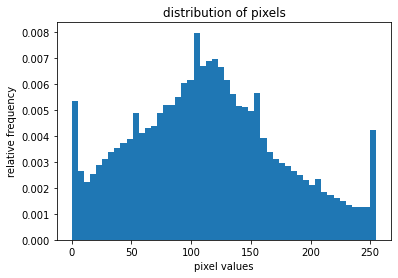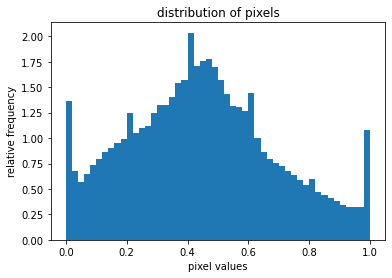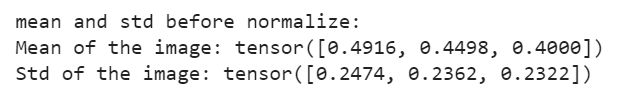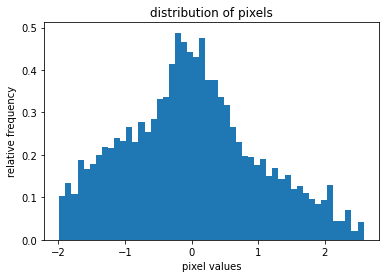Open in App
Not now

# How to normalize images in PyTorch ?

• Difficulty Level : Basic
• Last Updated : 06 Jun, 2022

Image transformation is a process to change the original values of image pixels to a set of new values. One type of transformation that we do on images is to transform an image into a PyTorch tensor. When an image is transformed into a PyTorch tensor, the pixel values are scaled between 0.0 and 1.0. In PyTorch, this transformation can be done using torchvision.transforms.ToTensor(). It converts the PIL image with a pixel range of [0, 255] to a PyTorch FloatTensor of shape (C, H, W) with a range [0.0, 1.0].

The normalization of images is a very good practice when we work with deep neural networks. Normalizing the images means transforming the images into such values that the mean and standard deviation of the image become 0.0 and 1.0 respectively. To do this first the channel mean is subtracted from each input channel and then the result is divided by the channel standard deviation.

`output[channel] = (input[channel] - mean[channel]) / std[channel]`

## Why should we normalize images?

Normalization helps get data within a range and reduces the skewness which helps learn faster and better. Normalization can also tackle the diminishing and exploding gradients problems.

## Normalizing Images in PyTorch

Normalization in PyTorch is done using torchvision.transforms.Normalize(). This normalizes the tensor image with mean and standard deviation.

Syntax: torchvision.transforms.Normalize()

Parameter:

• mean: Sequence of means for each channel.
• std: Sequence of standard deviations for each channel.
• inplace: Bool to make this operation in-place.

Returns: Normalized Tensor image.

### Approach:

We will perform the following steps while normalizing images in PyTorch:

• Load and visualize image and plot pixel values.
• Transform image to Tensors using torchvision.transforms.ToTensor()
• Calculate mean and standard deviation (std)
• Normalize the image using torchvision.transforms.Normalize().
• Visualize normalized image.
• Calculate mean and std after normalize and verify them.

Input image:Load the above input image using PIL. We are using the above Koala.jpg image in our program. And plot the pixel values of the image.

## Python3

 `# python code to load and visualize``# an image` `# import necessary libraries``from` `PIL ``import` `Image``import` `matplotlib.pyplot as plt``import` `numpy as np` `# load the image``img_path ``=` `'Koala.jpg'``img ``=` `Image.``open``(img_path)` `# convert PIL image to numpy array``img_np ``=` `np.array(img)` `# plot the pixel values``plt.hist(img_np.ravel(), bins``=``50``, density``=``True``)``plt.xlabel(``"pixel values"``)``plt.ylabel(``"relative frequency"``)``plt.title(``"distribution of pixels"``)`

Output:We find that pixel values of RGB image range from 0 to 255.

### Transforming images to Tensors using torchvision.transforms.ToTensor()

Convert the PIL image to a PyTorch tensor using ToTensor() and plot the pixel values of this tensor image. We define our transform function to convert the PIL image to a PyTorch tensor image.

## Python3

 `# Python code for converting PIL Image to``# PyTorch Tensor image and plot pixel values` `# import necessary libraries``import` `torchvision.transforms as transforms``import` `matplotlib.pyplot as plt` `# define custom transform function``transform ``=` `transforms.Compose([``    ``transforms.ToTensor()``])` `# transform the pIL image to tensor``# image``img_tr ``=` `transform(img)` `# Convert tensor image to numpy array``img_np ``=` `np.array(img_tr)` `# plot the pixel values``plt.hist(img_np.ravel(), bins``=``50``, density``=``True``)``plt.xlabel(``"pixel values"``)``plt.ylabel(``"relative frequency"``)``plt.title(``"distribution of pixels"``)`

Output:We find that pixel values of tensor image range from 0.0 to 1.0. We notice that the pixel distributions of RBG and tensor image look the same but differ in the pixel values range.

### Calculating mean and standard deviation (std)

We calculate the mean and std of the image.

## Python3

 `# Python code to calculate mean and std``# of image` `# get tensor image``img_tr ``=` `transform(img)` `# calculate mean and std``mean, std ``=` `img_tr.mean([``1``,``2``]), img_tr.std([``1``,``2``])` `# print mean and std``print``(``"mean and std before normalize:"``)``print``(``"Mean of the image:"``, mean)``print``(``"Std of the image:"``, std)`

Output:Here we calculated the mean and std of the image for all three channels Red, Green, and Blue. These values are before normalization.  We will use these values to normalize the image. We will compare these values with those after normalization.

### Normalizing the images using torchvision.transforms.Normalize()

To normalize the image, here we use the above calculated mean and std of the image. We can also use the mean and std of the ImageNet dataset if the image is similar to ImageNet images. The mean and std of ImageNet are: mean = [0.485, 0.456, 0.406] and std = [0.229, 0.224, 0.225]. If the image is not similar to ImageNet, like medical images, then it is always advised to calculate the mean and std of the dataset and use them to normalize the images.

## Python3

 `# python code to normalize the image`  `from` `torchvision ``import` `transforms` `# define custom transform``# here we are using our calculated``# mean & std``transform_norm ``=` `transforms.Compose([``    ``transforms.ToTensor(),``    ``transforms.Normalize(mean, std)``])` `# get normalized image``img_normalized ``=` `transform_norm(img)` `# convert normalized image to numpy``# array``img_np ``=` `np.array(img_normalized)` `# plot the pixel values``plt.hist(img_np.ravel(), bins``=``50``, density``=``True``)``plt.xlabel(``"pixel values"``)``plt.ylabel(``"relative frequency"``)``plt.title(``"distribution of pixels"``)`

Output:We have normalized the image with our calculated mean and std. The above output shows the distribution of the pixel values of the normalized image.  We can notice the difference between pixel distributions of tensor image (before normalize) and of normalized image.

### Visualizing the normalized image

Now visualize the normalized image.

## Python3

 `# Python Code to visualize normalized image` `# get normalized image``img_normalized ``=` `transform_norm(img)` `# convert this image to numpy array``img_normalized ``=` `np.array(img_normalized)` `# transpose from shape of (3,,) to shape of (,,3)``img_normalized ``=` `img_normalized.transpose(``1``, ``2``, ``0``)` `# display the normalized image``plt.imshow(img_normalized)``plt.xticks([])``plt.yticks([])`

Output:There are clear differences, we can notice, between the input image and normalized image.

### Calculating the mean and std after normalize

We calculate the mean and std again for normalized images/ dataset. Now after normalization, the mean should be 0.0, and std be 1.0.

## Python3

 `# Python code to calculate mean and std``# of normalized image` `# get normalized image``img_nor ``=` `transform_norm(img)` `# cailculate mean and std``mean, std ``=` `img_nor.mean([``1``,``2``]), img_nor.std([``1``,``2``])` `# print mean and std``print``(``"Mean and Std of normalized image:"``)``print``(``"Mean of the image:"``, mean)``print``(``"Std of the image:"``, std)`

Output:Here we find that after normalization the values of mean and std are 0.0 and 1.0 respectively. This verifies that after normalize the image mean and standard deviation becomes 0 and 1 respectively.

My Personal Notes arrow_drop_up